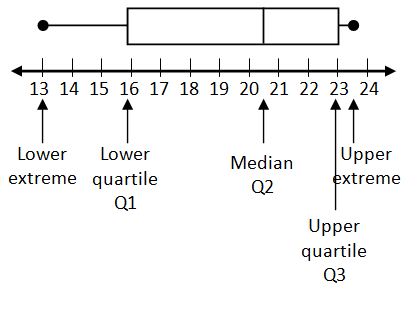Bar-type Graph

My Definition

Key Characteristics

The following are true of a bar-type graph:

• Bars of the same width are divided into countable sections to represent the frequency of data values in a data set.

• Each section represents one data point.

• Data may be numerical or categorical.

• A bar-type graph allows comparisons between and among quantities to be made.

Example

Vehicles Noted in Parking Lot
represents a bar-type graph of data.

Vehicles Noted in Parking LotNon-example

This is an example of a box-and-whisker plot.TEKS: 1(8)(B), 1(8)(C)